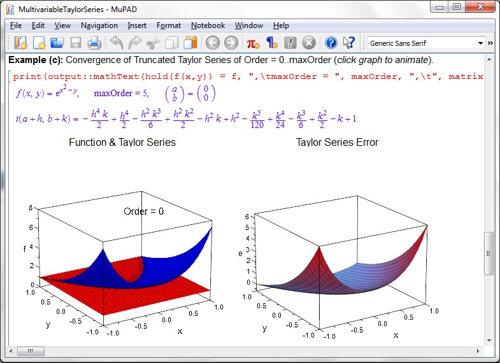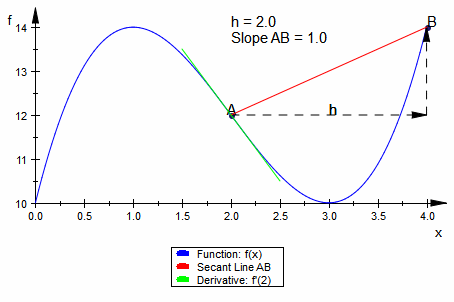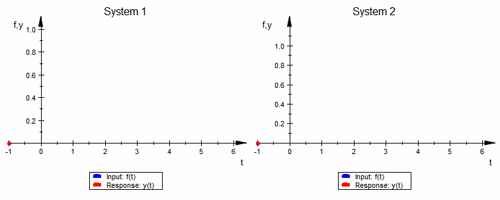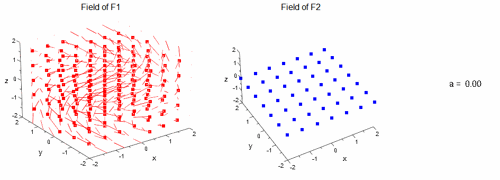## File Exchange Pick of the WeekOur best user submissions

Jiro's pick this week is MathExplorer by Martin Brown. Since MATLAB is, first and foremost, a numerical computation package, we haven't picked too many entries that use symbolic capabilities. Well, here it is, and it's quite impressive! Symbolic Math Toolbox™ extends MATLAB by providing functions for solving and manipulating symbolic expressions. In R2007b, we introduced a notebook interface called the MuPAD Notebook App. It provides an interactive environment for performing symbolic calculations and creating a dynamic document. MathExplorer is a set of hyperlinked MuPAD notebooks (43 total) for teaching mathematical concepts that are typically taught in the first and second year of college. With his notebooks, the students can learn the concepts interactively by running dynamic examples and experimenting with different parameters.The concepts that are covered are:
• Vectors
• Complex Numbers
• Differentiation
• Integration
• Taylor Series
• Multivariable Calculus
• Ordinary Differential Equations
• Laplace Transform
• Vector Calculus
• Linear Algebra
It also contains a few application examples for some of the topics. Many of his notebooks contain animated graphs to better convey the concepts. For example, you can learn about the concept of differentiation:Or see how first and second order systems respond to a step input:Or visualize a 3D vector field:Comments Students out there, learn about mathematical concepts in a fun, interactive way using MathExplorer! If you're a professor, give this a try in your courses! Let us know what you think here or leave a comment for Martin.

Published with MATLAB® R2012b

|